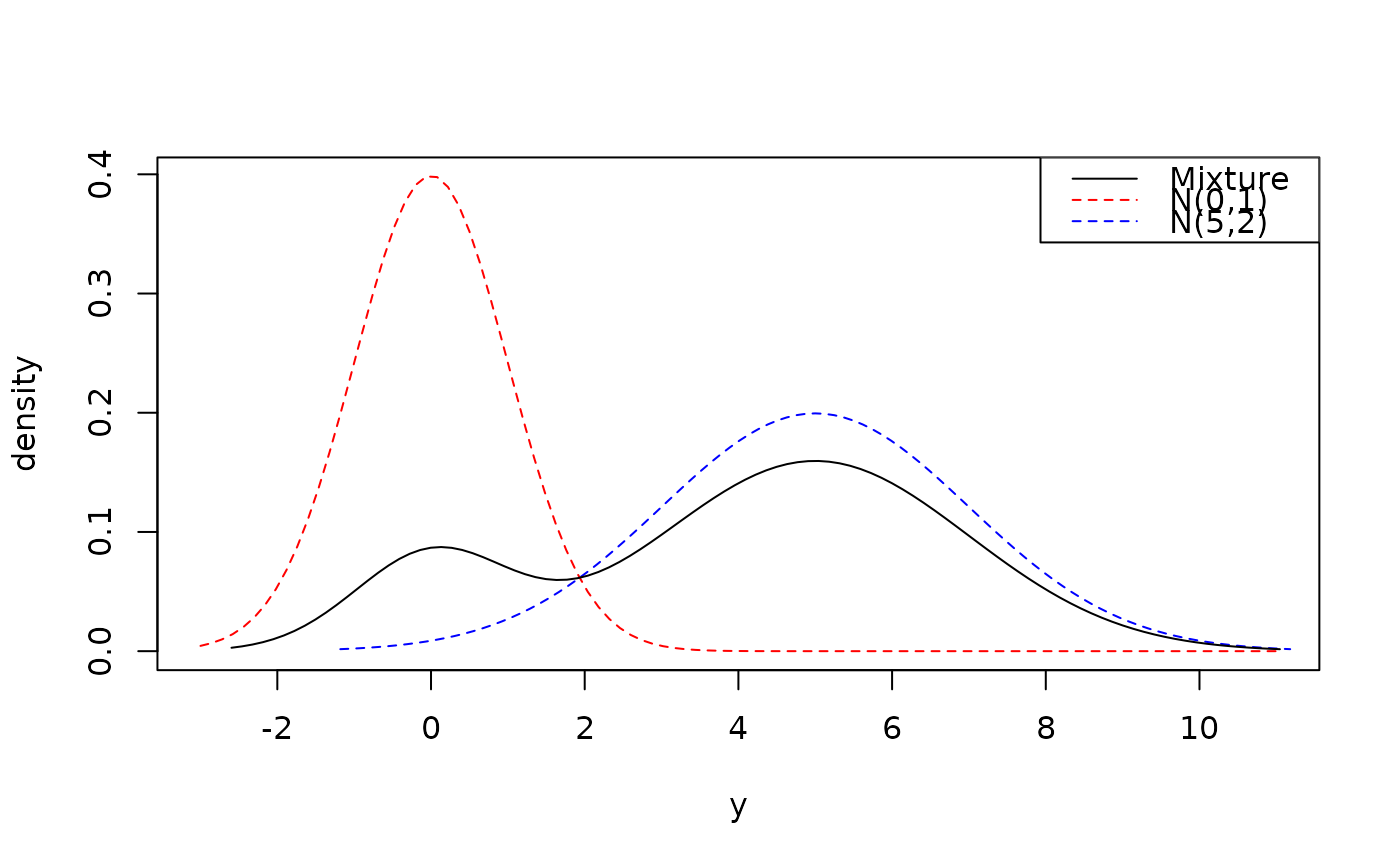Create a mixture distribution.

mix(..., weights = 1, na.rm = FALSE)

## Arguments

... Distribution objects to mix. Vector of weights corresponding to the distributions; or, single numeric for equal weights. Remove distributions corresponding to NA weights? Default is FALSE.

## Value

A mixture distribution -- an empty distribution if any weights are NA and na.rm = FALSE, the default.

## Examples

a <- dst_norm(0, 1)
b <- dst_norm(5, 2)
m1 <- mix(a, b, weights = c(1, 4))
plot(m1)
#> Warning: This function doesn't work properly yet!#> Warning: This function doesn't work properly yet!#> Warning: This function doesn't work properly yet!#> Warning: This function doesn't work properly yet!variable(m1)
#>  "continuous"
c <- stepdst(0:6)
m2 <- mix(a, b, c, weights = c(0.2, 0.5, 0.3))
plot(m2, n = 1001)
#> Warning: Density function does not exist. Plotting cdf instead.#> Warning: This function doesn't work properly yet!#> Warning: This function doesn't work properly yet!variable(m2)
#>  "mixed"